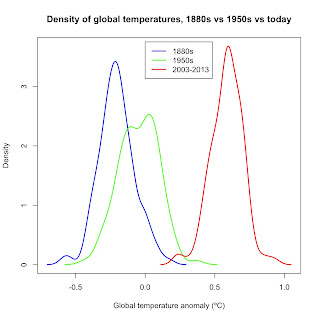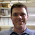### Shifting bell curves

One of the signatures of global warming is a shift in the frequency of global temperatures toward warmer temperatures.  One great way to visualize this shift in frequency is to plot out the densities of temperatures over several time periods.  For example, here is the density of global temperatures from 1880-1889, 1950-1959, and June 2003-May 2013:Data: GISS.  Baseline: 1951-1980 average (14.00ºC).
You can clearly see how the average global temperature has shifted toward the right over the past 133 years.  What is today the average isn't really in the range of possible temperatures in the 1880s and even the 1950s.  We can easily test how far things have shifted by using z-scores.

Z-scores are one of the simplest statistical tests both to calculate and interpret.  The formula is
where x = the data point you're interested in, x̄ = the average, and s = standard deviation.

What z-scores tell us is how many standard deviations any one data point is above or below the average.  We can then use the normal distribution to calculate the chances any data point will be that many standard deviations away from the average.   Using the 1880s average anomaly (x̄ = -0.2111ºC) and standard deviation (s = 0.1271ºC), I calculated the chances of global temperature during a single month matching the average for the 1950s (x = -0.0455ºC) and the last ten years (x = 0.5803ºC) if no global warming had occurred.  The results?

 Time periods z-score Probability 1950s - 1880s 4.48 1 in 270,270.3 Last 10 - 1880s 6.22 0

Mind you, this is the z-score of any one month in thd 1880s matching what is today the average.  Today's average has shifted so far above the temperatures of the 1880s that the chances of any one month then matching today's average is zero.  Now does that mean that we won't ever see months wherein global average temperatures are similar to those of the 1880s and 1950s?  Not yet.  The coldest months of today still overlap the warmest months of the 1880s and 1950s, as the graph shows.  So we could see a global monthly temperature today that is similar to months in those decades—if you want to call a very cold month of today matching a very warm month of yesteryear "similar."

In short, the global temperature distribution has clearly shifted to the right.  And it's that shift in distribution that is the main signature of global warming.

Edit (5/4/2017): As requested, I have written a post containing my code to create a bell curve graph as I did in this post. You can find it here: http://environmentalforest.blogspot.com/2017/05/r-code-for-shifting-bell-curves.html

1.Hey Jim,
thank you for the interesting post! I would be interested in the code you used to produce the graph. I would guess you used the np package for R, however I was not successful in replicating the graph.
Thanks!

1.I'll put the full code in a post but for right now, try using the density function.

2.If you need your ex-girlfriend or ex-boyfriend to come crawling back to you on their knees (no matter why you broke up) you got to watch this video
right away...

(VIDEO) Win your ex back with TEXT messages?